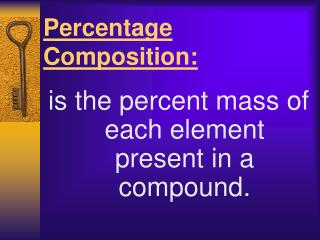DownloadDownload PresentationPercentage Composition:

Percentage Composition:

Télécharger la présentationPercentage Composition:

- - - - - - - - - - - - - - - - - - - - - - - - - - - E N D - - - - - - - - - - - - - - - - - - - - - - - - - - -
Presentation Transcript

1. Percentage Composition: is the percent mass of each element present in a compound.

2. Percent Composition Can be calculated if given: the chemical formula OR masses of elements in compound

3. By Chemical Formula % mass = molar mass of an element X 100% total molar mass of the compound

4. Example: What is the % composition of CaCO3? CHEMICAL FORMULA • Step 1: Find the molar mass of CaCO3 : Ca x 1 = 40.1 g/mol • C x 1 = 12.0 g/mol • O x 3 = 48.0 g/mol • CaCO3 = 100.1 g/mol

5. Step 2: Find the % composition: % Ca = 40.1 g/mol x 100 % = 40.1 % Ca 100.1 g/mol % C = 12.0 g/mol x 100% = 12.0 % C 100.1 g/mol % O = 48.0 g/mol x 100 % = 48.0 % O 100.1 g/mol

6. Example: Calculate the percent composition of the compounds that is formed from this reaction: 29.0g of Ag combines completely with 4.30g of S. STEP 1: find the total mass of the elements. 29.0g + 4.30g = 33.30g STEP 2: find the % composition Ag = 29.0g x 100% = 87.1% 33.30g S = 4.30g x 100% = 12.9% 33.30g Masses of elements in compound

7. Try These: • Find the percent composition of KMnO4. • Calculate the % composition of the compound that results from 9.03g Mg reacting completely with 3.48g N.

8. ANSWERS: • 1) K = 24.7% Mn = 34.8% O = 40.5% 2) Mg = 72.2% N = 27.8%

9. Do Problem 17 on page 131 • Do Problem 18 & 19 on page 133 CHECK YOUR ANSWERS!

10. Example 2 How much carbon is present in 15.2 g of carbon dioxide gas? % carbon = 12.0 g/mol x 100 % = 27.3 % 44.0 g/mol Xg carbon = 15.2 g of CO2 X 27.3 g C 100. g CO2 = 4.15 g of Carbon

11. Percent Composition • Can be used to:  calculate the mass of elements in a compound  determine the empirical formula of a compound  determine the molecular formula of a compound

12. Empirical Formula • shows the simplest mole ratio of the elements. • CO is a 1:1 ratio of carbon to oxygen • H2O is a 2:1 ratio • CO2 is a 1:2 ratio • Empirical formulas can’t be reduced.

13. Molecular Formula • shows the actual number of atoms in a molecule. • The molecular formula for hydrogen peroxide is H2O2. Its empirical formula would be HO. • Often the molecular formula is the same as the empirical formula: H2O, CO2

14. Empirical? • CH4O • yes, cannot be reduced further • C2H6 • no, empirical would be CH3 • C3H10O • yes • C6H6O2 • no. What would empirical be? • C3H3O

15. Calculating Empirical Formulas • A chemist with an unknown compound can easily figure out its percent composition, but it is much more meaningful to know its formula. • EXAMPLE: What is the empirical formula for a compound that is 25.9% nitrogen and 74.1% oxygen?

16. Method • Write the mass (g) of each element in the compound. So….we assume that it is a 100g sample: 25.9% N = 25.9g 74.1% O = 74.1g

17. 2. Convert the mass of each element to moles, by dividing by the molar mass. • N = 25.9g = 1.85 mol 14.0g/mol • O = 74.1g = 4.63 mol 16.0g/mol

18. Calculate the simplest whole number ratio by dividing the number of moles by the smallest number of moles. 1.85:4.63 = 1 : 2.5 1.85 1.85 (If the result is not within 0.1 of a whole number, multiply all numbers by a whole number) 2 ( 1 : 2.5) = 2 : 5

19. Write the empirical formula using the numbers you obtained. N2 O5 NOTE: • For inorganic compounds, write the most positive element first. • For organic compounds, write C first, H second and all others alphabetically.

20. A special present just for you…….. • Page 135, Problems #20 & 21 • Check your answers

21. Molecular Formula Given the empirical formula and the gram formula mass (gfm) OR Given the percent composition and the gram formula mass (gfm)

22. Example #1 Calculate the molecular formula for NaO having a gfm of 78g.  Determine the efm (empirical formula mass). NaO = 23.0g + 16.0g = 39.0 • Divide the efm into the gfm. 78.0 = 2 39.0 • This is the conversion factor used to determine the molecular formula. Na2O2

23. Example #2 Find the molecular formula for a compound having a composition of 58.8% C, 9.8% H and 31.4% O and a gmm of 102g/mol. • Determine the mass of each component. C = 102g/mol x 58.8% = 60.0g/mol H = 102g/mol x 9.8% = 10.0g/mol O = 102g/mol x 31.4% = 32.0g/mol

24.  convert to moles C = 60.0g/mol = 5 12.0g H = 10.0g/mol = 10 1.0g O = 32.0g/mol = 2 16.0g

25.  Use moles as subscripts for components of compound C5H10O2 • Check the gmm of this compound…does it equal 102.0g/mol? • 5(12.0) + 10(1.0) + 2(16.0) = 102.0g/mol • YES!

26. And Now….. • Oh Yeah! And there’s more… • Page 136, Problems #22 & 23

27. Now Try page 139, #41 44